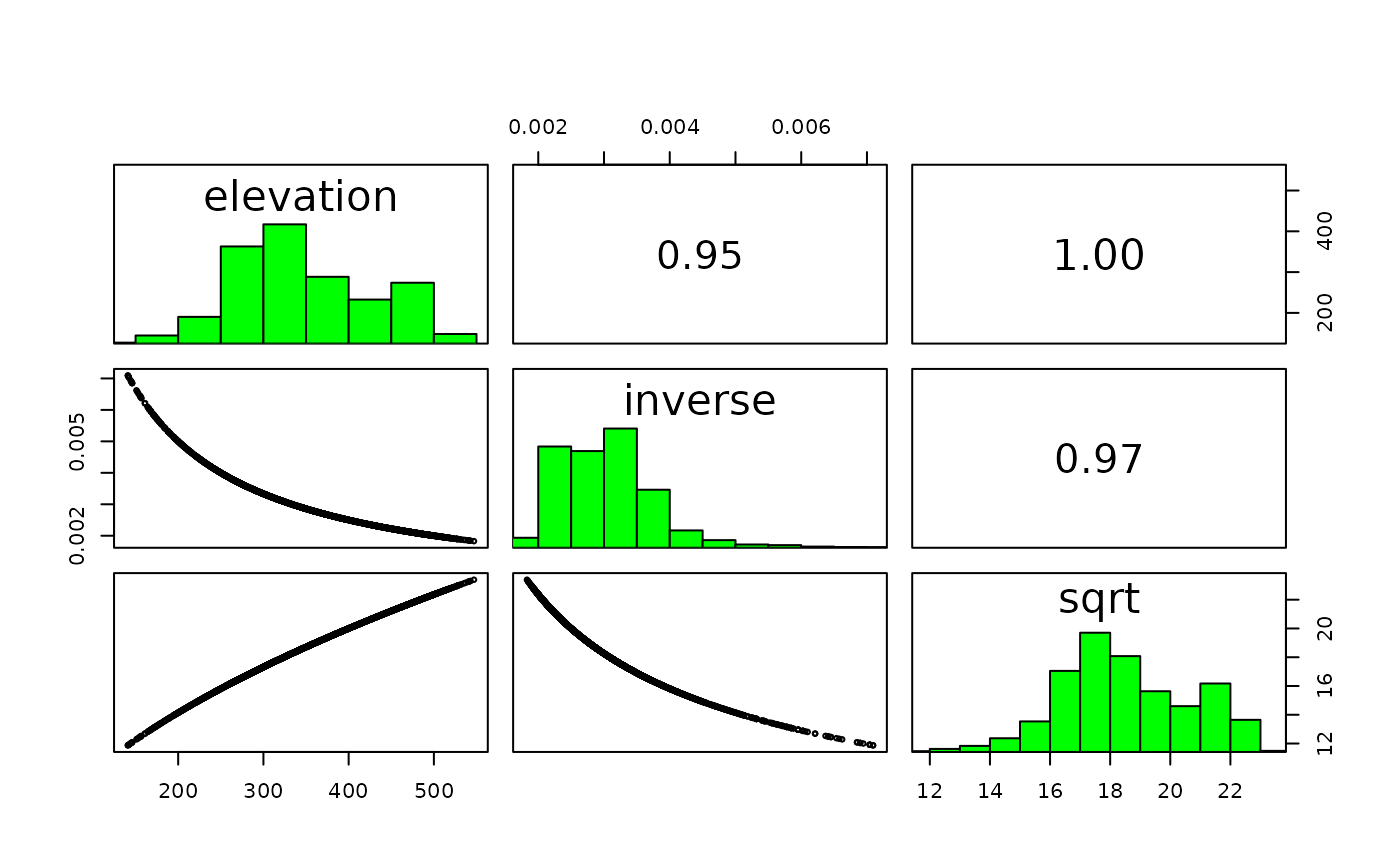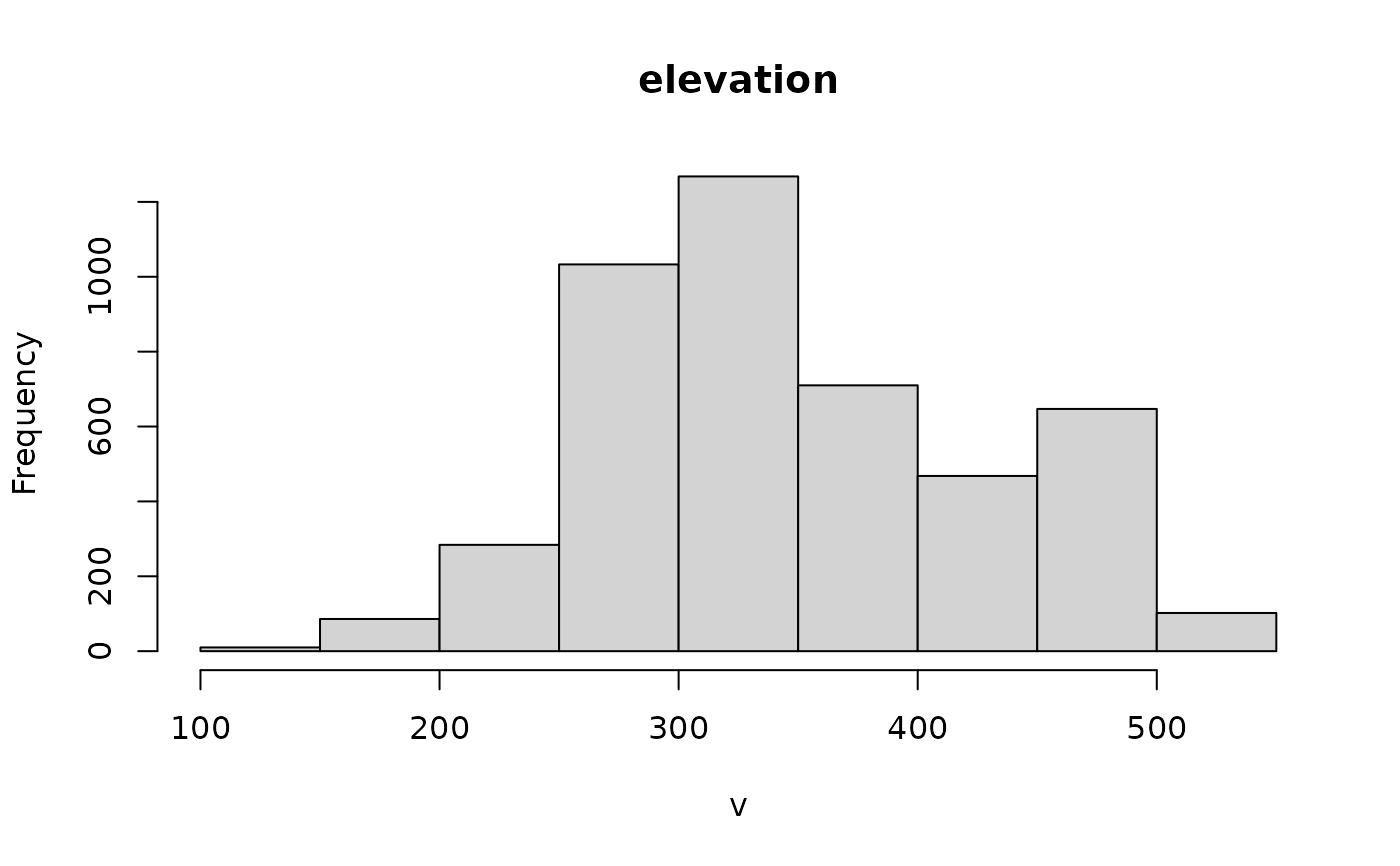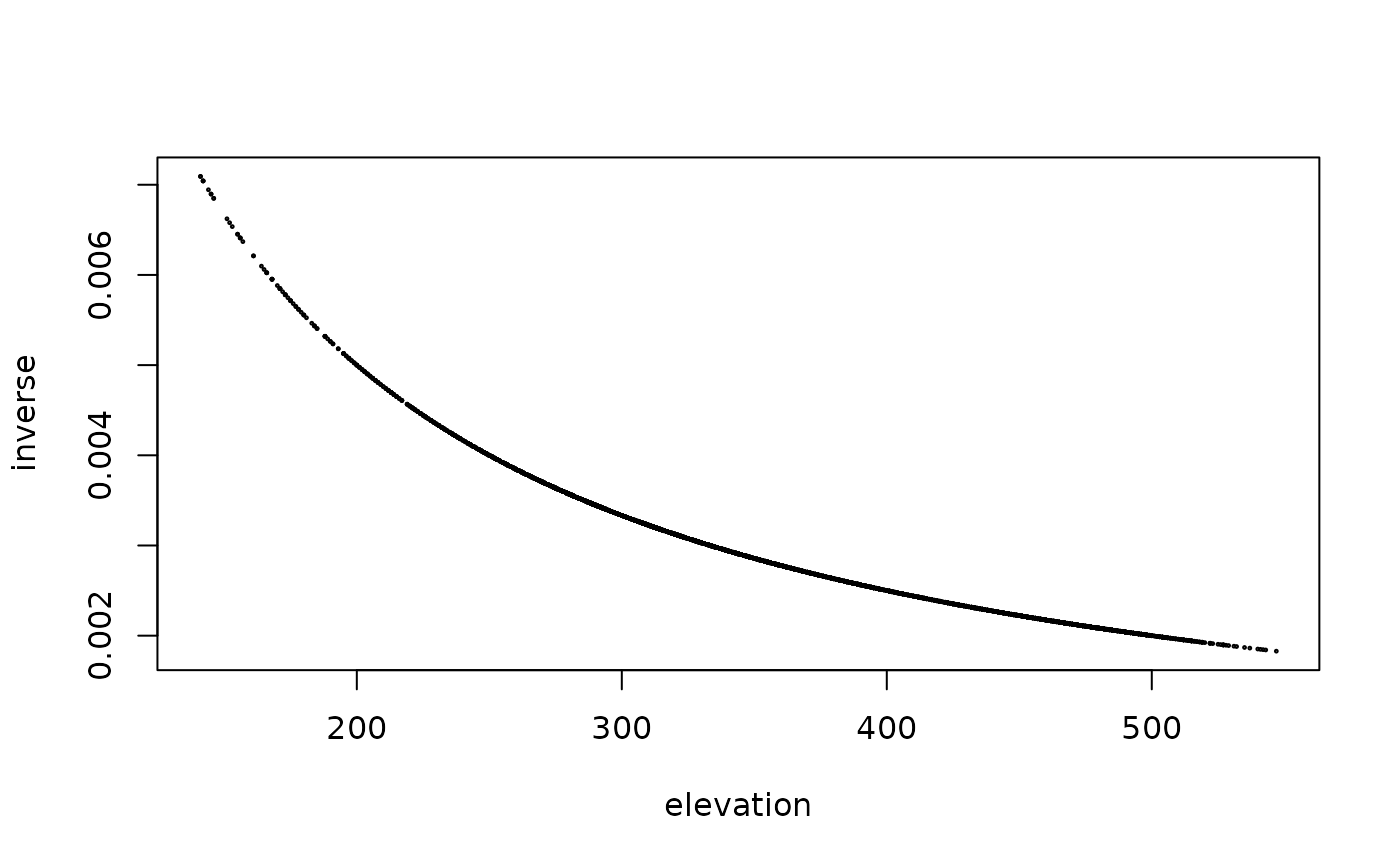Pair plots of layers in a SpatRaster. This is a wrapper around graphics function pairs.

# S4 method for SpatRaster
pairs(x, hist=TRUE, cor=TRUE, use="pairwise.complete.obs", maxcells=100000, ...)

## Arguments

x

SpatRaster

hist

logical. If TRUE a histogram of the values is shown on the diagonal

cor

logical. If TRUE the correlation coefficient is shown in the upper panels

use

argument passed to the cor function

maxcells

integer. Number of pixels to sample from each layer of a large SpatRaster

...

boxplot, hist

## Examples

r <-rast(system.file("ex/elev.tif", package="terra"))
s <- c(r, 1/r, sqrt(r))
names(s) <- c("elevation", "inverse", "sqrt")
pairs(s)# to make indvidual histograms:
hist(r)# or scatter plots:
plot(s[], s[])